# Hash function

Mar. 7, 2019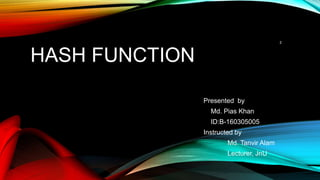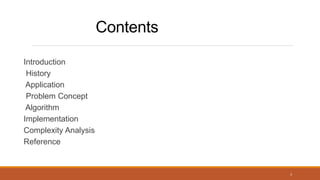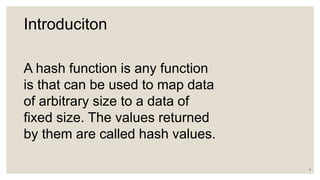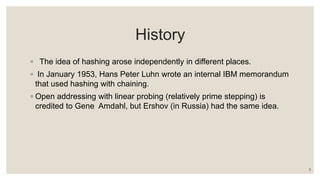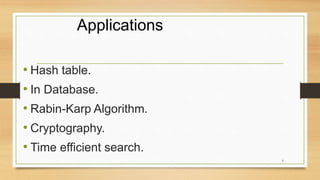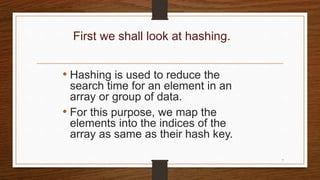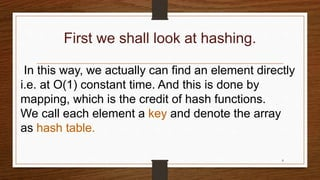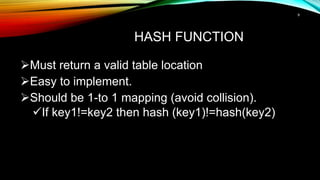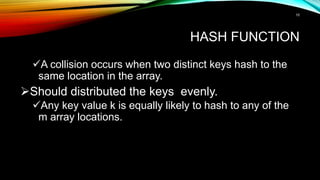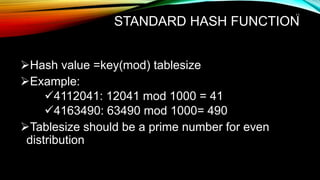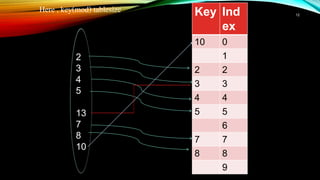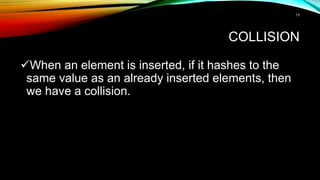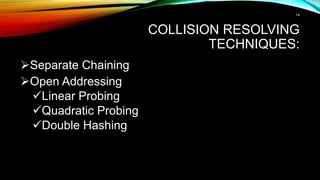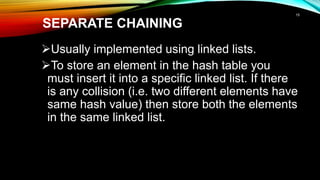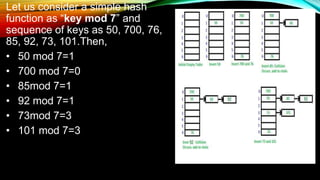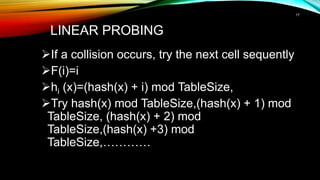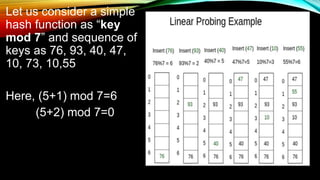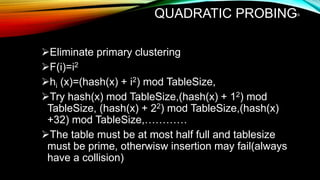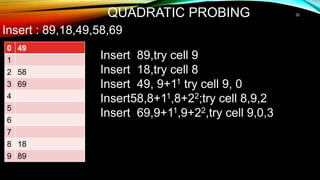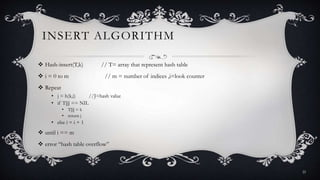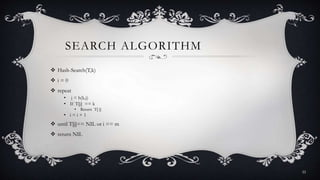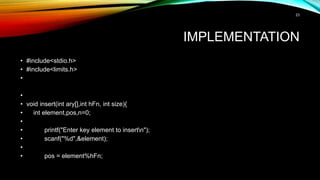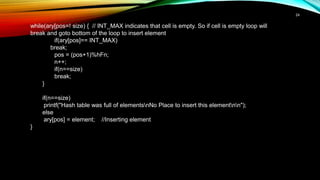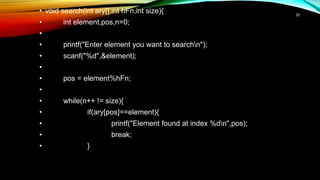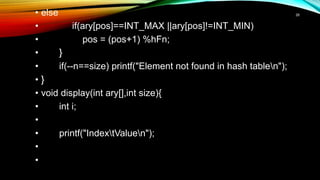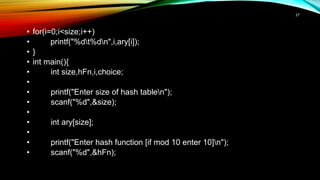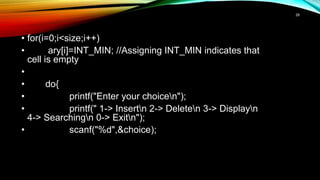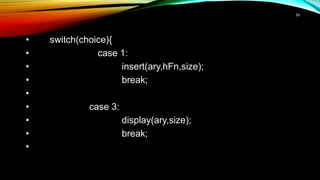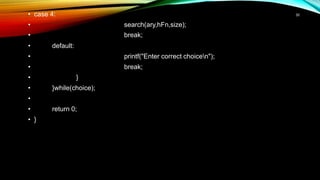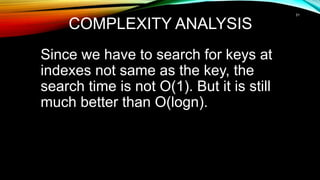1 of 33

### Hash function

• 1. WELCOME TO OUR PRESENTATION PRESENTED BY MD PIAS KHAN & MAHDEE TAHMID INSTRUCTED BY TANVIR AHAMMED LECTURER, JAGANNATH UNIVERSITY 1
• 2. HASH FUNCTION Presented by Md. Pias Khan ID:B-160305005 Instructed by Md. Tanvir Alam Lecturer, JnU 2
• 4. Introduciton A hash function is any function is that can be used to map data of arbitrary size to a data of fixed size. The values returned by them are called hash values. 4
• 5. History ◦ The idea of hashing arose independently in different places. ◦ In January 1953, Hans Peter Luhn wrote an internal IBM memorandum that used hashing with chaining. ◦ Open addressing with linear probing (relatively prime stepping) is credited to Gene Amdahl, but Ershov (in Russia) had the same idea. 5
• 6. Applications • Hash table. • In Database. • Rabin-Karp Algorithm. • Cryptography. • Time efficient search. 6
• 7. First we shall look at hashing. • Hashing is used to reduce the search time for an element in an array or group of data. • For this purpose, we map the elements into the indices of the array as same as their hash key. 7
• 8. First we shall look at hashing. In this way, we actually can find an element directly i.e. at O(1) constant time. And this is done by mapping, which is the credit of hash functions. We call each element a key and denote the array as hash table. 8
• 9. HASH FUNCTION Must return a valid table location Easy to implement. Should be 1-to 1 mapping (avoid collision). If key1!=key2 then hash (key1)!=hash(key2) 9
• 10. HASH FUNCTION A collision occurs when two distinct keys hash to the same location in the array. Should distributed the keys evenly. Any key value k is equally likely to hash to any of the m array locations. 10
• 11. STANDARD HASH FUNCTION Hash value =key(mod) tablesize Example: 4112041: 12041 mod 1000 = 41 4163490: 63490 mod 1000= 490 Tablesize should be a prime number for even distribution 11
• 12. Here , key(mod) tablesize 2 3 4 5 13 7 8 10 Key Ind ex 10 0 1 2 2 3 3 4 4 5 5 6 7 7 8 8 9 12
• 13. COLLISION When an element is inserted, if it hashes to the same value as an already inserted elements, then we have a collision. 13
• 14. COLLISION RESOLVING TECHNIQUES: Separate Chaining Open Addressing Linear Probing Quadratic Probing Double Hashing 14
• 15. SEPARATE CHAINING Usually implemented using linked lists. To store an element in the hash table you must insert it into a specific linked list. If there is any collision (i.e. two different elements have same hash value) then store both the elements in the same linked list. 15
• 16. Let us consider a simple hash function as “key mod 7” and sequence of keys as 50, 700, 76, 85, 92, 73, 101.Then, • 50 mod 7=1 • 700 mod 7=0 • 85mod 7=1 • 92 mod 7=1 • 73mod 7=3 • 101 mod 7=3 16
• 17. LINEAR PROBING If a collision occurs, try the next cell sequently F(i)=i hi (x)=(hash(x) + i) mod TableSize, Try hash(x) mod TableSize,(hash(x) + 1) mod TableSize, (hash(x) + 2) mod TableSize,(hash(x) +3) mod TableSize,………… 17
• 18. Let us consider a simple hash function as “key mod 7” and sequence of keys as 76, 93, 40, 47, 10, 73, 10,55 Here, (5+1) mod 7=6 (5+2) mod 7=0 18
• 19. QUADRATIC PROBING Eliminate primary clustering F(i)=i2 hi (x)=(hash(x) + i2) mod TableSize, Try hash(x) mod TableSize,(hash(x) + 12) mod TableSize, (hash(x) + 22) mod TableSize,(hash(x) +32) mod TableSize,………… The table must be at most half full and tablesize must be prime, otherwisw insertion may fail(always have a collision) 19
• 20. QUADRATIC PROBING 0 49 1 2 58 3 69 4 5 6 7 8 18 9 89 Insert : 89,18,49,58,69 Insert 89,try cell 9 Insert 18,try cell 8 Insert 49, 9+11 try cell 9, 0 Insert58,8+11,8+22;try cell 8,9,2 Insert 69,9+11,9+22,try cell 9,0,3 20
• 21. INSERT ALGORITHM  Hash-insert(T,k) // T= array that represent hash table  i = 0 to m // m = number of indices ,i=look counter  Repeat • j = h(k,i) //J=hash value • if T[j] == NIL • T[j] = k • return j • else i = i + 1  until i == m  error “hash table overflow” 21
• 22. SEARCH ALGORITHM  Hash-Search(T,k)  i = 0  repeat • j = h(k,i) • If T[j] == k • Return T[ j] • i = i + 1  until T[j]== NIL or i == m  return NIL 22
• 23. IMPLEMENTATION • #include<stdio.h> • #include<limits.h> • • • void insert(int ary[],int hFn, int size){ • int element,pos,n=0; • • printf("Enter key element to insertn"); • scanf("%d",&element); • • pos = element%hFn; 23
• 24. while(ary[pos=! size) { // INT_MAX indicates that cell is empty. So if cell is empty loop will break and goto bottom of the loop to insert element if(ary[pos]== INT_MAX) break; pos = (pos+1)%hFn; n++; if(n==size) break; } if(n==size) printf("Hash table was full of elementsnNo Place to insert this elementnn"); else ary[pos] = element; //Inserting element } 24
• 25. • void search(int ary[],int hFn,int size){ • int element,pos,n=0; • • printf("Enter element you want to searchn"); • scanf("%d",&element); • • pos = element%hFn; • • while(n++ != size){ • if(ary[pos]==element){ • printf("Element found at index %dn",pos); • break; • } 25
• 26. • else • if(ary[pos]==INT_MAX ||ary[pos]!=INT_MIN) • pos = (pos+1) %hFn; • } • if(--n==size) printf("Element not found in hash tablen"); • } • void display(int ary[],int size){ • int i; • • printf("IndextValuen"); • • 26
• 27. • for(i=0;i<size;i++) • printf("%dt%dn",i,ary[i]); • } • int main(){ • int size,hFn,i,choice; • • printf("Enter size of hash tablen"); • scanf("%d",&size); • • int ary[size]; • • printf("Enter hash function [if mod 10 enter 10]n"); • scanf("%d",&hFn); 27
• 28. • for(i=0;i<size;i++) • ary[i]=INT_MIN; //Assigning INT_MIN indicates that cell is empty • • do{ • printf("Enter your choicen"); • printf(" 1-> Insertn 2-> Deleten 3-> Displayn 4-> Searchingn 0-> Exitn"); • scanf("%d",&choice); 28
• 29. • switch(choice){ • case 1: • insert(ary,hFn,size); • break; • • case 3: • display(ary,size); • break; • 29
• 30. • case 4: • search(ary,hFn,size); • break; • default: • printf("Enter correct choicen"); • break; • } • }while(choice); • • return 0; • } 30
• 31. COMPLEXITY ANALYSIS Since we have to search for keys at indexes not same as the key, the search time is not O(1). But it is still much better than O(logn). 31
• 32. REFERENCES  https://en.wikipedia.org/wiki/Hash_table  Introduction to Algorithms, Third Edition by By Thomas H. Cormen, Charles E. Leiserson, Ronald L. Rivest and Clifford Stein 32
• 33. • Hoping that you have no question to us, we conclude our presentation. Yet if someone wishes to embarrass us, other than our honorable teacher , I shall not bother to answer my dear mates.